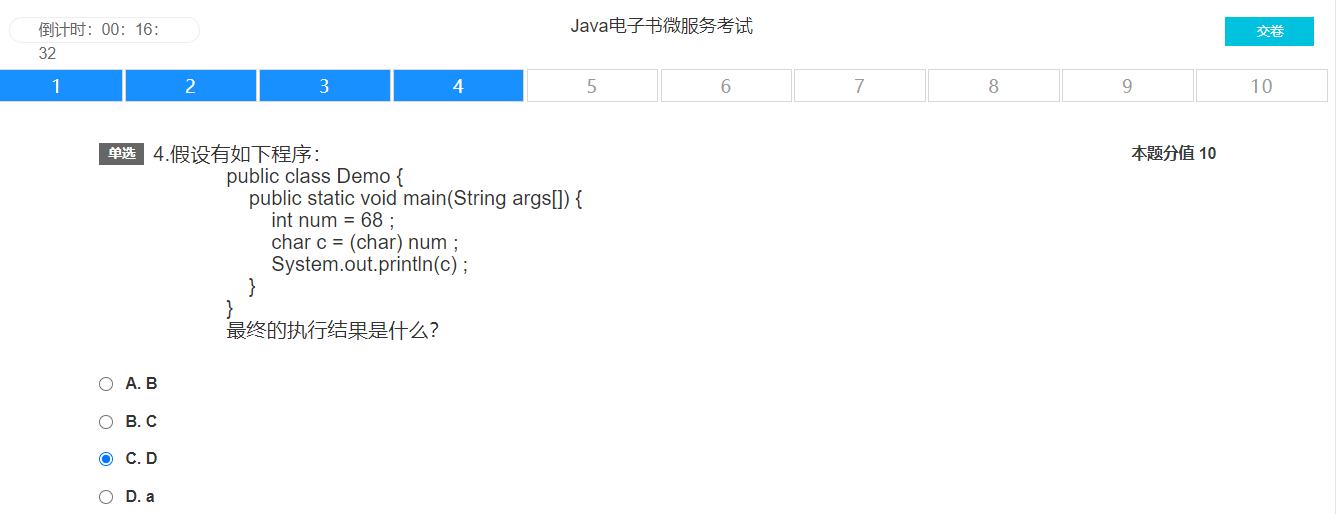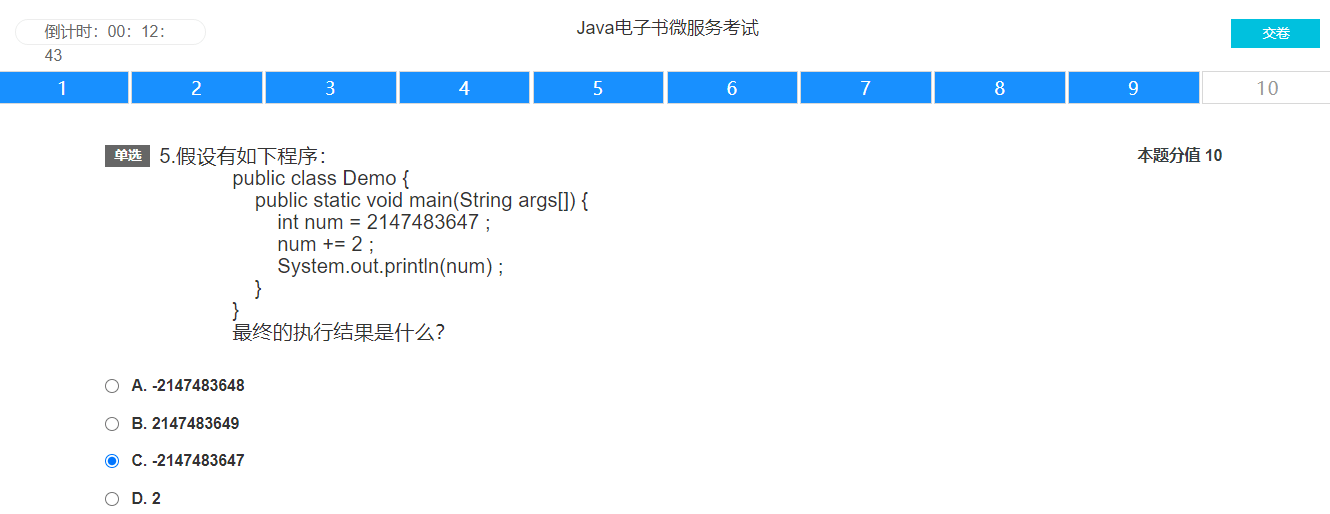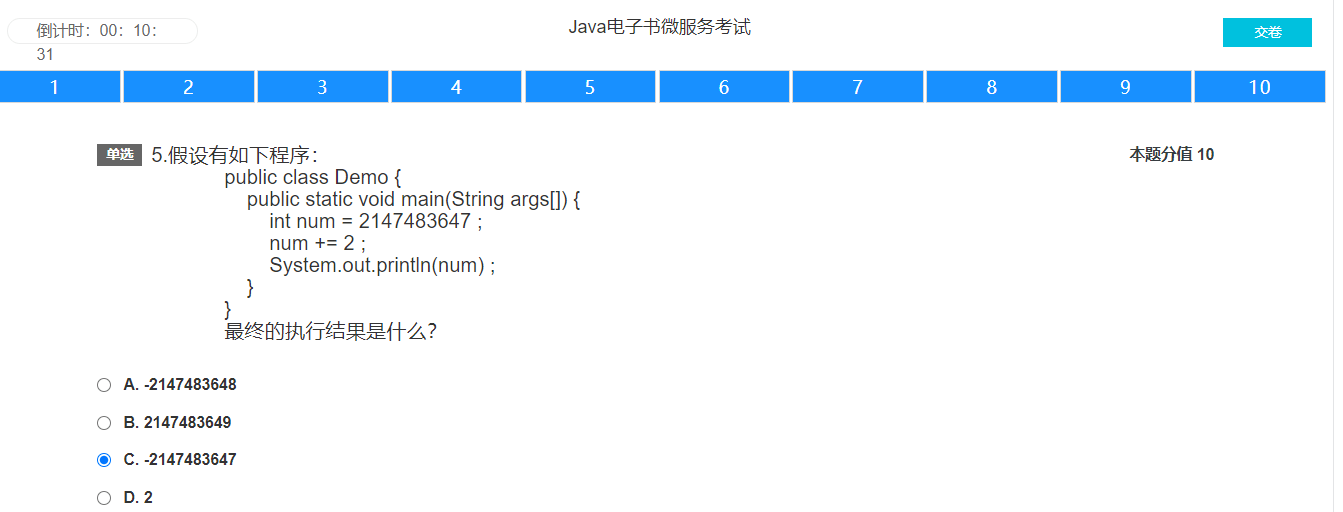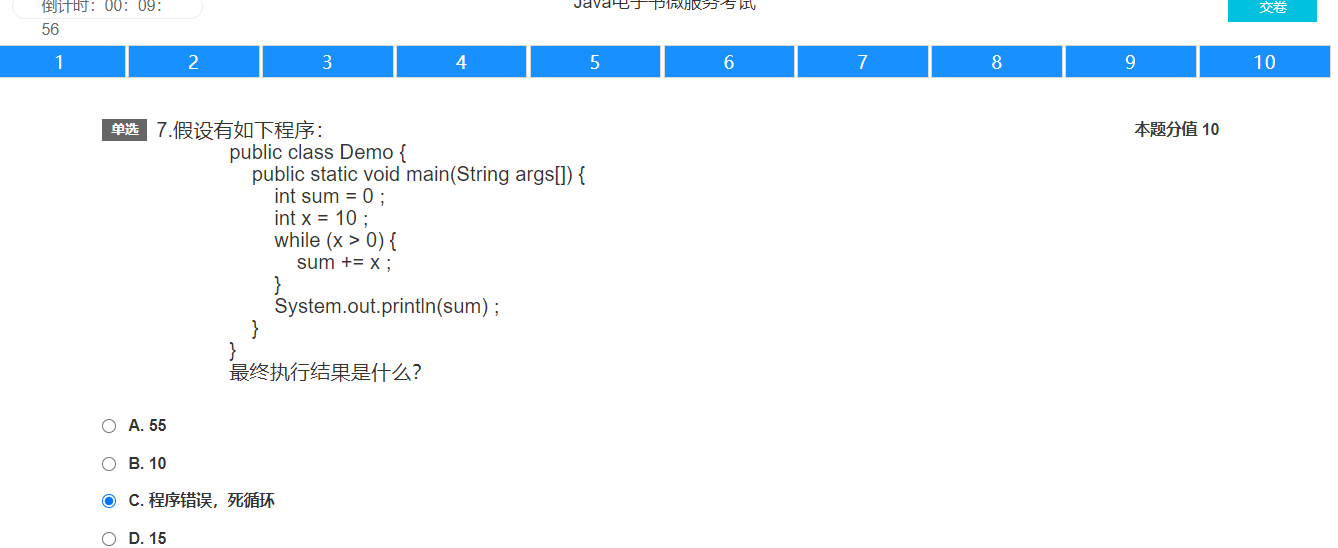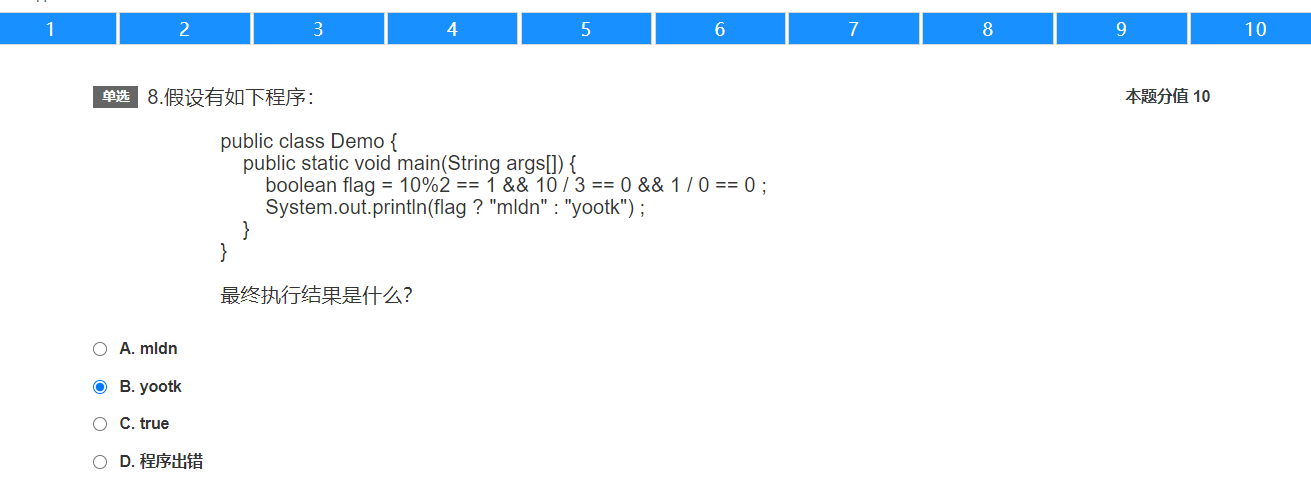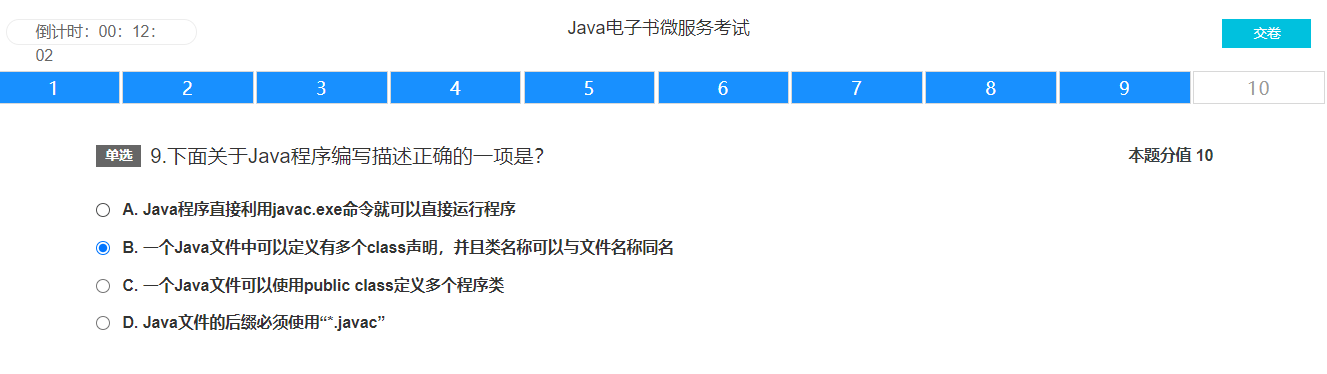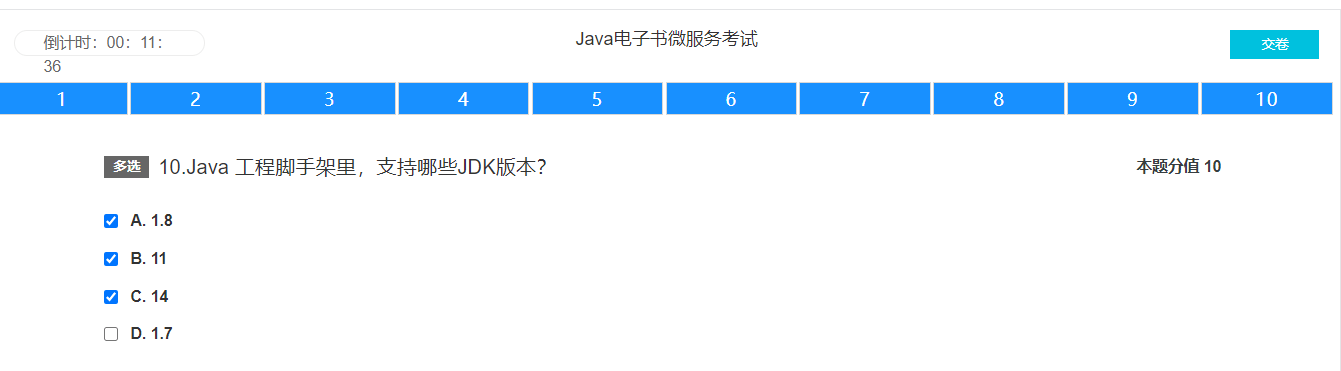# 阿里云x intelliJ联名悠悠球微服务自测答案

2020年9月15日其他活动

## 100分答案：

### 1、假设有如下程序：

```public class Demo {
public static void main(String args[]) {
int num = 2147483647;
long temp = num + 2L;
System.out.println(num);
}
}```

A .-2147483648
B .2147483649
C .2147483647
D .2

### 2、现在有一个方法：public static int info（int x，double y），下面那个方法是对本方法的正确重载？（C）

A.public static int infos（int x，inty）；
B.public static void info（int x，double y）；
C.public static int info（int x，inty）；
D.public static void infos（int x，inty）；

### 3、下面那一项关于基本数据类型的描述是正确的？（A）

A .boolean数据类型只有true和false两种取值
B .使用long可以保存小数
C .float数据类型可以保存的数据范围比double数据范围要大
D .byte数据类型可以正常保存200这个数字

### 4、假设有如下程序：

```public class Demo {
public static void main(String args[]) {
boolean flag = 10 % 2 == 1 && 10 / 3 == 0 && 1 / 0 == 0;
System.out.println(flag ? "mldn" : "yootk");
}
}```

A .mldn
B .yootk
C .true
D .程序出错

A . // 注释
B . – 注释
C . /注释…/
D . / 注释…*/

A.CLASSPATH
B.GC
C.TMP
D.CPU

### 7、假设有如下程序：

```public class Demo {
public static void main(String args[]) {
char c = 'A';
int num = 10;
switch (c) {
case 'B':
num++;
case 'A':
num++;
case 'Y':
num++;
break;
default:
num--;
}
System.out.println(num);
}
}```

A、11
B、13
C、12
D、10

### 8、假设有如下程序：

```public class Demo {
public static void main(String args[]) {
String str = "";
for (int x = 0; x < 5; x++) {
str += x;
}
System.out.println(str);
}
}```

A .01234
B .10
C .14
D .25

### 9、假设有如下程序：

```public class Demo {
public static void main(String args[]) {
int x = 10;
double y = 20.2;
long z = 10L;
String str = "" + x + y * z;
System.out.println(str);
}
}```

A .10202.0
B .0212.0
C .302.0
D .1020.210

### 10、假设有如下程序：

```public class Demo {
public static void main(String args[]) {
int sum = 0;
int x = 10;
while (x > 0) {
sum += x;
}
System.out.println(sum);
}
}```

A .55
B .10
C .程序错误，死循环
D .15

### 11、下面关于Java的特点不正确的一项是？（B）

A .Java具备跨平台性，可以在任意的操作系统间进行移植
B .Java编写的程序可以直接解释执行，属于解释型的编程语言类型
C .Java中具备垃圾收集机制，这样在用户编写代码中无须处理手工处理内存空间的释放操作
D.Java EE企业级开发是在Java SE基础之上的扩展应用

### 12、假设有如下程序：

```public class Demo {
public static void main(String args[]) {
int num = 68;
char c = (char) num;
System.out.println(c);
}
}```

A .B
B . C
C . D
D . a

### 13、假设有如下程序：

```public class Demo {
public static void main(String args[]) {
int num = 50;
num = num++ * 2;
System.out.println(num);
}
}```

A .50
B .102
C .100
D .101

### 14、假设有如下程序：

```public class Demo {
public static void main(String args[]) {
System.out.println(inc(10) + inc(8) + inc(-10));
}

public static int inc(int temp) {
if (temp > 0) {
return temp * 2;
}
return -1;
}
}```

A .35
B .8
C .28
D .12

B .int
C .boolean
D .String

B.goto
C.enum
D.assert

### 17、假设有如下程序：

```public class Demo {
public static void main(String args[]) {
int num = 2147483647;
num += 2;
System.out.println(num);
}
}```

A .-2147483648
B .2147483649
C .-2147483647
D .2

### 18、下面的数据声明及赋值哪一个是正确的？（D）

A .float f = 1.3;
B .char c =
C .byte b = 257
D .int i = 10

### 19、下面关于Java程序编写描述正确的一项是？（B）

A .Java程序直接利用javac.exe命令就可以直接运行程序
B .一个Java文件中可以定义有多个class声明，并且类名称可以与文件名称同名
C .一个Java文件可以使用public class定义多个程序类
D .Java文件的后缀必须使用“*.javac”

A.void
B.int
C.public
D.static

## 90分答案：